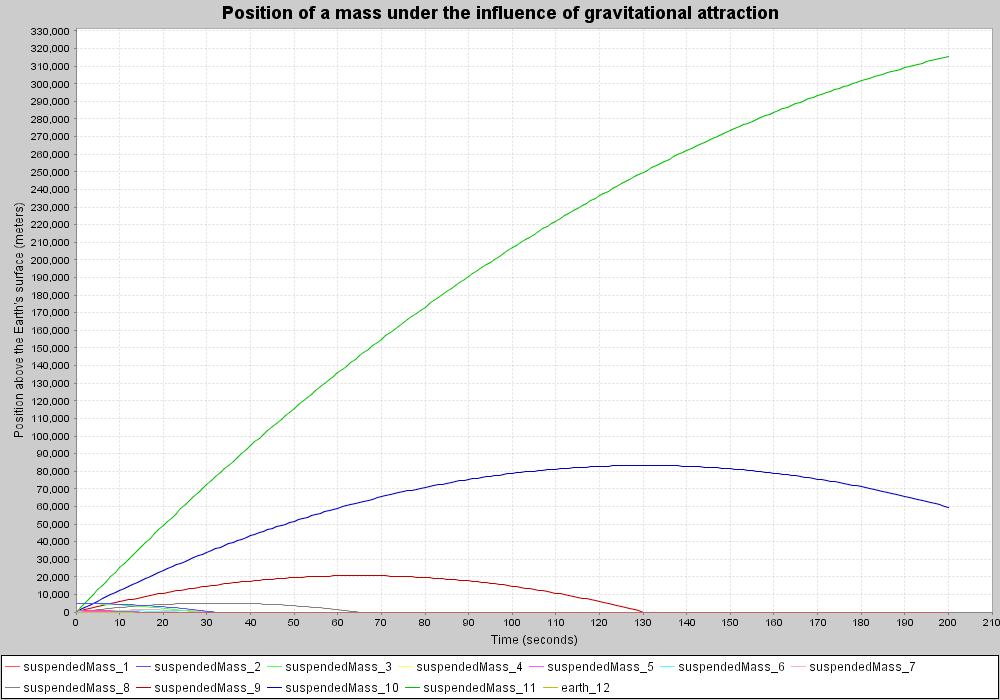# Dynamical System - Gravity 1 - Xholon App

## What is it

This model implements a dynamical system in which a small mass, given a variety of initial masses and velocities, is suspended above the surface of the Earth and acted upon by gravity. This demonstrates how to model dynamical systems using Xholon.

## How to use it

Run it:

1. Run the Java application through the Xhn GUI (org.primordion.xholon.app.Xhn.java), and select File --> Open --> dynsys --> Gravity1 --> Gravity1_xhn.xml.
2. Expand the Controller node in the tree.
3. Press the Start node.
4. If you have JFreeChart installed you should see a chart like the one below showing the Position series over time. To zoom in on a time period and range use the left mouse button to select the area of interest.
```

```## Things to notice

Because the vertical acceleration is constant, and the rate of change in vertical position is smooth and gradual, then the size of the time step interval is not especially critical in this application. The timeStepMultiplier constant, defined in XhGravity1.java has a value of 2 (IIntegration.M_2), and provides only a small correction to a value of 1 (IIntegration.M_1). A value of 1 is the same as not using Xholon's Euler-like numerical integration. A value of 2 means that each Xholon time step is broken into two finer intervals. In the limit, as the time step multiplier approaches infinity, then the actual values obtained during the simulation will approach the true value found in nature. In this application, a value of 1 or 2 may very well be good enough, depending on what you're trying to show. If you set the value to IIntegration.M_1024, then each time will be broken into 1024 intervals, which should produce a more accurate result, but at the cost of a longer processing time.

## Things to try

You can change the time period under observation by editing Gravity1_xhn.xml (param name="MaxProcessLoops" value="200"/>).

Set various initial positions and velocities by editing CompositeStructureHierarchy.xml.

## Extending the model

Try additional gravity curves, by cloning the existing ones.

## Xholon features

If you press "View --> Position of a mass under the influence of gravitational attraction:xYChart_24" before pressing "Controller --> Start", then you can observe the changes of the line chart at each time step.

## Credits and references

source: Speigel, M. (1967). Applied Differential Equations, 2nd ed. Englewood Cliffs: Prentice-Hall. p.64-66